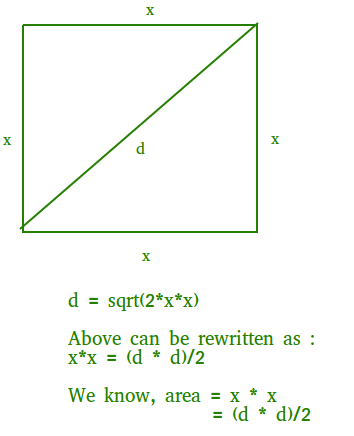# Area of a square from diagonal length

Given an number d which is length of diagonal of a square, find its area.
Examples:

```Input : d = 10
Output : Area = 50

Input : d = 12.2
Output : Area = 74.42
```

## Recommended: Please try your approach on {IDE} first, before moving on to the solution.

Area of a square can be computed as (d * d)/2. Please see below image for details.## C++

 `// C++ Program to find the area of square ` `// when its diagonal is given. ` `#include ` `using` `namespace` `std; ` ` `  `// Returns area of square from given ` `// diagonal ` `double` `findArea(``double` `d) ` `{ ` `    ``return` `(d * d) / 2.0; ` `} ` ` `  `// Driver Code ` `int` `main() ` `{ ` `    ``double` `d = 10; ` `    ``cout << (findArea(d)); ` `    ``return` `0; ` `} ` ` `  `// This code is contributed by ` `// Shivi_Aggarwal `

## C

 `// C Program to find the area of square ` `// when its diagonal is given. ` `#include ` ` `  `// Returns area of square from given ` `// diagonal ` `double` `findArea(``double` `d) ` `{ ` `    ``return` `(d * d) / 2; ` `} ` ` `  `// Driver function. ` `int` `main() ` `{ ` `    ``double` `d = 10; ` `    ``printf``(``"%.2f"``, findArea(d)); ` `    ``return` `0; ` `} `

## Java

 `// Java Program to find the area of square ` `// when its diagonal is given. ` ` `  `class` `GFG  ` `{ ` `    ``// Returns area of square from given ` `    ``// diagonal ` `    ``static` `double` `findArea(``double` `d) ` `    ``{ ` `        ``return` `(d * d) / ``2``; ` `    ``} ` `     `  `    ``// Driver code ` `    ``public` `static` `void` `main (String[] args)  ` `    ``{ ` `        ``double` `d = ``10``; ` `        ``System.out.println(findArea(d)); ` `    ``} ` `} ` ` `  `// This code is contributed by Anant Agarwal. `

## Python3

 `# Python3 Program to find ` `# the area of square ` `# when its diagonal is given. ` ` `  `# Returns area of square from given ` `# diagonal ` `def` `findArea(d): ` ` `  `    ``return` `(d ``*` `d) ``/` `2` ` `  `# Driver function. ` `d ``=` `10` `print``(``"%.2f"` `%` `findArea(d)) ` ` `  `# This code is contributed by ` `# Smitha Dinesh Semwal `

## C#

 `// C# Program to find the area of square ` `// when its diagonal is given. ` `using` `System; ` ` `  `class` `GFG  ` `{ ` `    ``// Returns area of square from given ` `    ``// diagonal ` `    ``static` `double` `findArea(``double` `d) ` `    ``{ ` `        ``return` `(d * d) / 2; ` `    ``} ` `     `  `    ``// Driver code ` `    ``public` `static` `void` `Main ()  ` `    ``{ ` `        ``double` `d = 10; ` `        ``Console.WriteLine(findArea(d)); ` `    ``} ` `} ` ` `  `// This code is contributed by vt_m. `

## PHP

 ` `

Output:

```50.00
```

Attention reader! Don’t stop learning now. Get hold of all the important DSA concepts with the DSA Self Paced Course at a student-friendly price and become industry ready.

My Personal Notes arrow_drop_upCheck out this Author's contributed articles.

If you like GeeksforGeeks and would like to contribute, you can also write an article using contribute.geeksforgeeks.org or mail your article to contribute@geeksforgeeks.org. See your article appearing on the GeeksforGeeks main page and help other Geeks.

Please Improve this article if you find anything incorrect by clicking on the "Improve Article" button below.

Improved By : vt_m, Shivi_Aggarwal